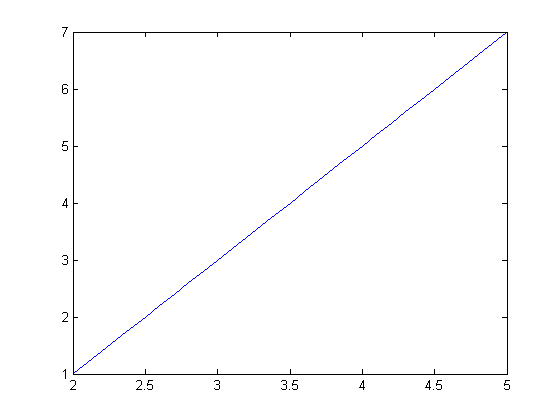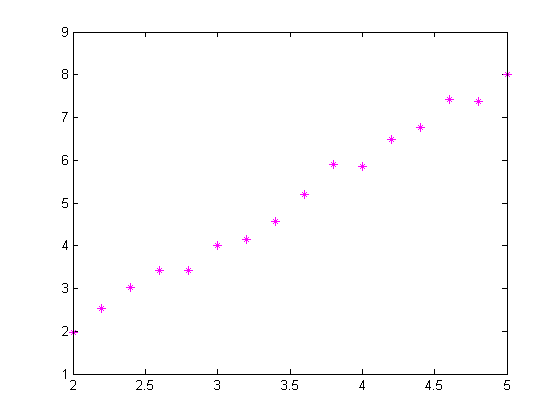## Loren on the Art of MATLABTurn ideas into MATLAB

One of the reasons I like using anonymous functions is because they allow me to express whatever function I want to use as a function of the relevant arguments in different contexts.

### Contents

#### Example

Here's a very general way I can write the code meant to generate points on a straight line.

straightline = @(X,slope,intercept) slope*X + intercept

straightline =
@(X,slope,intercept)slope*X+intercept


#### Choose a Particular Line to Plot

First let's choose a particular straight line segment. I start by defining the slope and intercept values.

m = 2;  % slope
b = -3; % intercept


#### Plot the Line

Now I can evaluate my function when I create the plot.

x = 2:0.1:5;
plot(x,straightline(x,m,b))#### Find Area Under the Line

Now let's find the area under a segment of this line, between two points. Since the function integral requires the integrand to be a function of one variable only, we can use the anonymous function myline, defined below, to create just such a representation.

lowerlimit = 2;
upperlimit = 5;


Create a function representing my particular line segment, by fixing those slope and intercept values, and creating a new function from the original one.

myline = @(x) straightline(x,m,b);


Use this new function, myline, as the integrand.

myarea = integral(myline, lowerlimit, upperlimit)

myarea =
12


#### Find Best Fit Line

Now suppose we have data, based on this line segment, that has some noise. And we would like to estimate the slope and intercept values.

m = 2;
b = -2;
t = (2:0.2:5)';
data = straightline(t,m,b) + 0.2*randn(size(t));
plot(t,data,'m*')Now I want slope and intercept to be my independent variables. I can use polyfit. Of course, I could use \ as well.

p = polyfit(t,data,1);


Now compare estimates for slope and intercept to original values.

comparison = [m, b; p]

comparison =
2           -2
2.094      -2.3638


Perhaps I instead want a function of the slope and intercept only. In that case, with data supplied, I can derive such a function from straightline. I can even get the values put into a

slopeIntercept = @(slope,intercept) straightline(data, slope, intercept);
slp = [1 4]; intcpt = [0 -5];
newline1 = @(X) straightline(X, slp(1), intcpt(1));
newline2 = @(X) straightline(X, slp(2), intcpt(2));


#### Anonymous Functions Allow Interface Flexibility

Using the same original function definition for straightline, I am able to mold the function for different purposes. In the first case, I turned my function of 3 variables into a function of one variable, with preset parameters. I could also use it to create a function of just slope and intercept, suitable for create line segments for different parameters at a later time.

In fact, if I was trying to use my straightline function with some other code that required lines to be defined by slope and intercept first, and then the abscissa, I can easily create a new interface for the line.

differentLine = @(slope, intercept, X) straightline(X, slope, intercept);


#### Have You Used Anonymous Functions to Alter the Interface?

I'd love to hear your thoughts here.

Published with MATLAB® 7.14

|